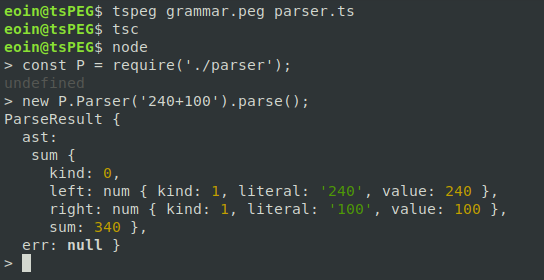# Vey.ie

Eoin Davey.
- MSc. Mathematics @ Universiteit van Amsterdam.
- Éireannach.

# tsPEG - The latest update

v1.3.0 of tsPEG has just been released! Check it out on Github or install it with npm install -g tspeg.

Below is a list of the latest new features:

## Computed Properties

As well as assigning parsing results to variables and storing them on the AST, tsPEG also allows you to create computed properties, which are fields on the AST that are computed when the parser is run.

Computed properties are added to a rule by appending a new expression after the rule description like

.<propertyname> = <type> { <code to calculate property> }


Let’s specify a small grammar to match simple addition expressions like “12+42”, “123+456” etc.

sum := left=num '\+' right=num
num := '[0-9]+'


We can add computed properties to this to compute the value of this sum at parse time, instead of writing our own function to do it after. First we add a computed property to num to store the value of the number:

sum := left=num '\+' right=num
num := literal='[0-9]+'
.value = number { return parseInt(this.literal); }


As you can see we’ve assigned the text match to the field literal, and added a computed property called value which is the literal field parsed as an integer.

Now we can add a computed property to sum to do the arithmetic, this is very simple

sum := left=num '\+' right=num
.sum = number { return this.left.value + this.right.value }
num := literal='[0-9]+'
.value = number { return parseInt(this.literal); }


We use the computed property of the num rule to compute our sum property. Let’s see this in action! Let’s try and parse the string “240+100”.As you can see the AST has a field called sum with the correct value of 340 in it. The left and right also have their computed value properties of 240 and 100.

The introduction of computed properties means that now you might want to import some types or functions into your parser. Luckily you can now specify a header in the file that will be inserted directly into the generated parser. Anything at the top of the grammar file between two lines of three dashes --- will be inserted straight into the generated parser, allowing you to write grammars like

---
import { myFunc, myType } from "./mypackage";
---
rule := hello='Hello World'
.value = myType { return myFunc(this.hello); }


## Position tracking

tsPEG now supports a special match expression “@” for storing the positions of matches. Using @ you can store the current position of the parser.

### Example

Returning to our addition of two numbers from earlier, which matches “12+56”, “123+456” etc.

sum := left=num '\+' right=num
num := '[0-9]+'


If we want to store the location of the “+” operator we can use the @ match like this:

sum := left=num pluspos=@ '\+' right=num
num := '[0-9]+'


This stores the position of the parser into the AST before the ‘+’ symbol, the generated interface for the AST looks like:

interface sum {
left: string;
pluspos: PosInfo;
right: string;
}